• 对1000万个数排序
千次阅读
2017-09-12 21:48:39
//因为BitSet中可以存true/false,而且是按位存储，所以在数据量很大的时候，合理的使用BitSet可以节省很大的内存空间，
//提高程序的运算效率。

// 下面是我使用Bitset和Arrays工具类进行排序的测试类

public class BitSetSort {

public static void main(String[] args) {
//		String sortNums = sortNums(generateNumber(10000000));
sortNums1(generateNumber(10000000));
//		System.out.println(sortNums);
}

// 初始化一千万整数
private static int[] generateNumber(int size){
long start = System.currentTimeMillis();
System.out.println("开始生成数据");
int[] nums = new int[size];
for(int i=0;i<size;i++){
nums[i] = RandomUtils.nextInt(0, size);
}
System.out.println("生成数据完成,耗时:"+(System.currentTimeMillis()-start)+"毫秒");
return nums;
}

// 使用BitSet进行排序
private static String sortNums(int[] nums){
long start = System.currentTimeMillis();
System.out.println("开始排序");
int len = nums.length;
StringBuilder sb = new StringBuilder();
BitSet bitSet = new BitSet(len);
bitSet.set(0, len, false);
for(int i=0;i<len;i++){
bitSet.set(nums[i], true);
}
for(int i=0;i<len;i++){
if(bitSet.get(i)){
sb.append(i).append(",");
}
}
System.out.println("排序完成,耗时:"+(System.currentTimeMillis()-start)+"毫秒");
return sb.toString();
}

// 使用Arrays工具类进行排序
private static int[] sortNums1(int[] nums){
long start = System.currentTimeMillis();
System.out.println("开始排序");
Arrays.sort(nums);
System.out.println("排序完成,耗时:"+(System.currentTimeMillis()-start)+"毫秒");
return nums;
}

}

结果：

1、使用BitSet排序

开始生成数据
生成数据完成,耗时:172毫秒
开始排序
排序完成,耗时:429毫秒

2、使用Arrays工具类排序

开始生成数据
生成数据完成,耗时:172毫秒
开始排序
排序完成,耗时:1078毫秒

更多相关内容
• 1000万整数中寻找最大的100个数，最优的算法是建一大小为100的小顶堆（堆排序需要建立完全二叉树），先取出前100个数并构建小顶堆，然后遍历数据与小顶堆的堆顶相比，比堆顶小直接丢弃，比堆顶大则替换堆顶，并...

这一段时间写毕业设计，遇到一些大数据的情况，想起之前和同学讨论的最优算法，写一下相关思路。

在1000万整数中寻找最大的100个数，最优的算法是建一个大小为100的小顶堆（堆排序需要建立完全二叉树），先取出前100个数并构建小顶堆，然后遍历数据与小顶堆的堆顶相比，比堆顶小直接丢弃，比堆顶大则替换堆顶，并重新构建这个堆。

### 堆排序

先介绍一下完全二叉树：除了最后一层之外的其他每一层都被完全填充，并且所有结点都保持向左对齐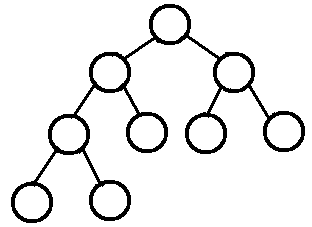堆排序：堆排序是将数据看成是完全二叉树、根据完全二叉树的特性来进行排序的一种算法

• 最小堆要求节点元素都不大于其左右孩子
• 那么处于最小堆的根节点的元素一定是这个堆中的最小值

模拟一次最小堆排序的过程：

假如有

        int[] arr = new int[]{3,6,8,7,0,1,10,4,2};

第一步：建立完全二叉树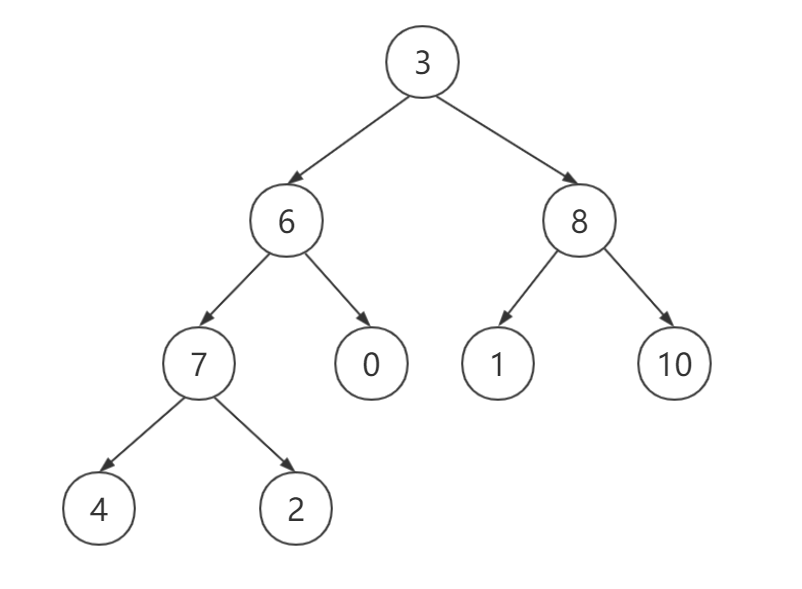从上图可以看出，第n个节点的左子节点为2*n+1，右子节点为2*n+2

第二步：从 arr.length/2 - 1 就是最后一个叶子节点的父节点开始，比较其左右子节点，找到最小的，并与其交换，一直到根节点：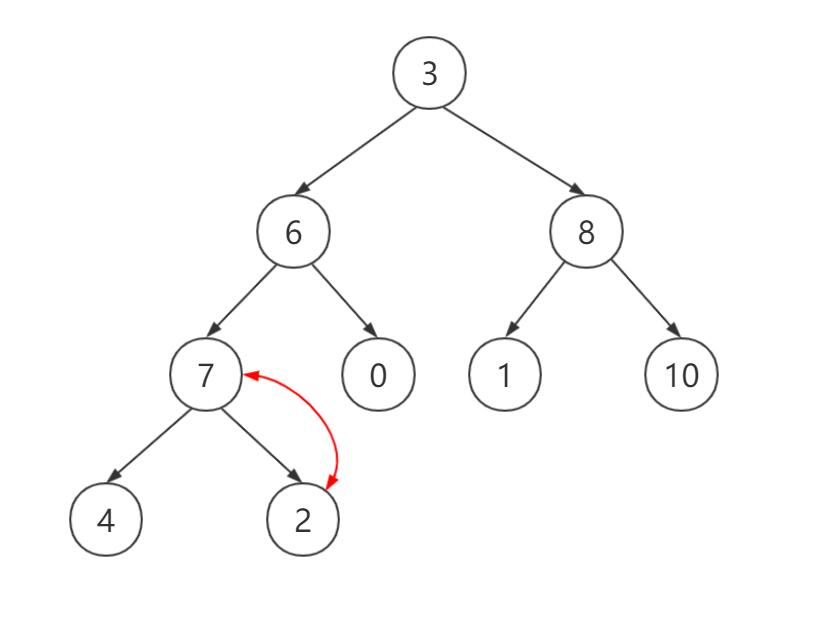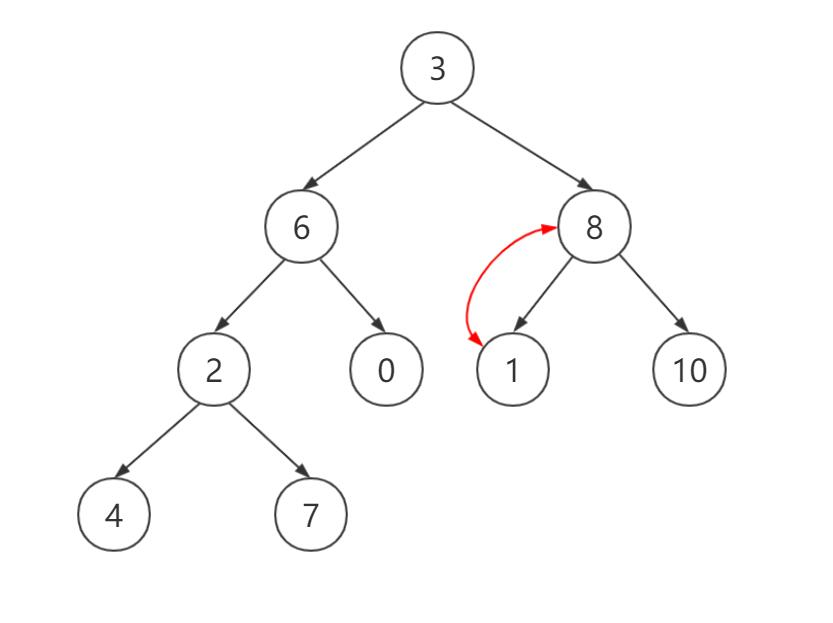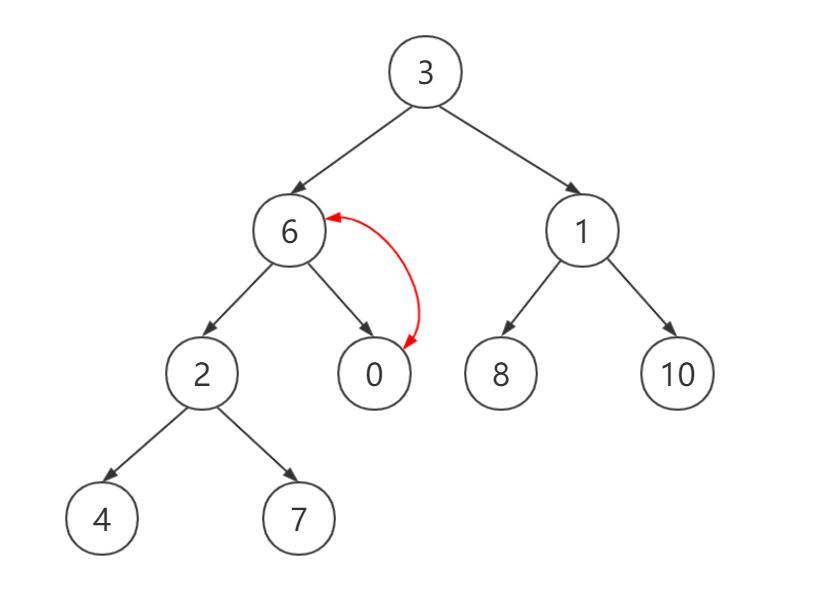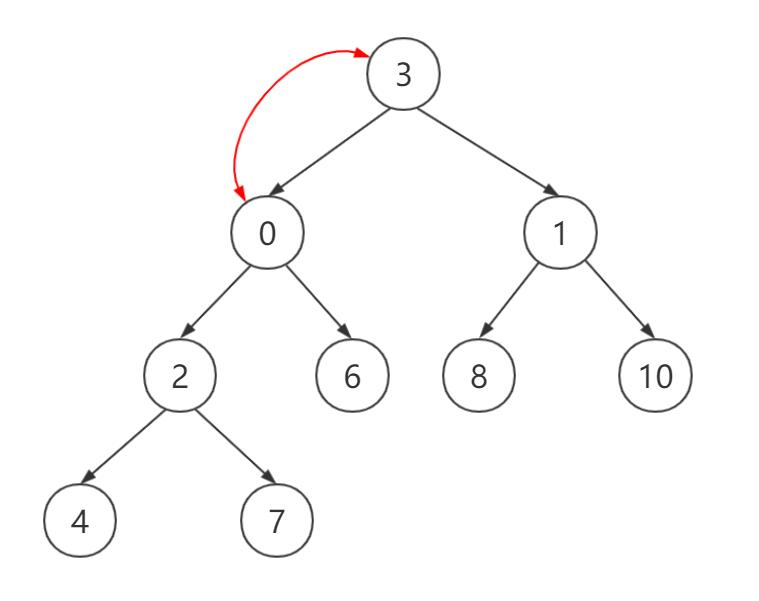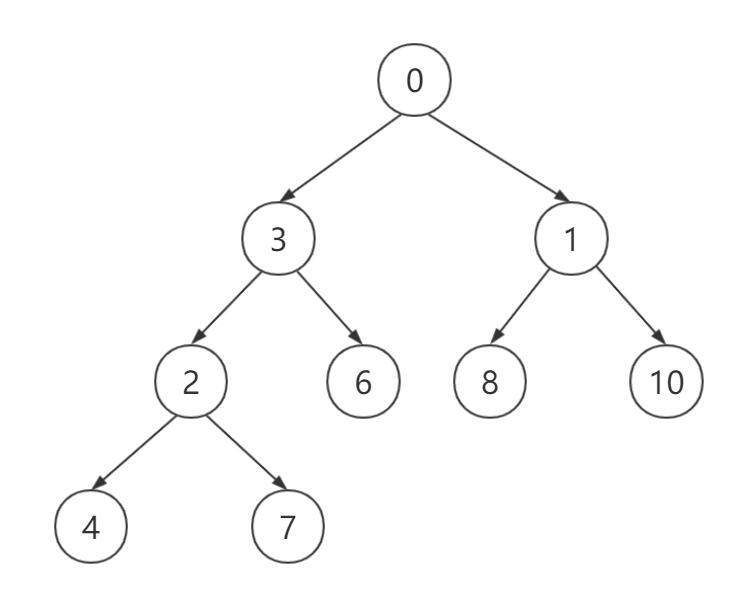这样一个最小堆就建立完成。

相关代码（java）：

    /**
* 建堆
*
* @param arr          看作是完全二叉树
* @param currentNode 当前父节点位置
* @param size            节点总数
*/
public class HeapSortTest {
public static void main(String[] args) {
int[] arr = new int[]{3,6,8,7,0,1,10,4,2};
int start = arr.length/2 -1;
for (int i = start; i >= 0 ; i--){
minHeap(arr, arr.length, i);
}
System.out.println(Arrays.toString(arr));
}
public static void minHeap(int []arr, int size, int currentNode){
//左子树和右字数的位置
int leftNode = currentNode * 2 + 1;
int rightNode = currentNode * 2 + 2;
//把当前父节点位置看成是最小的
int min = currentNode;

//比较左右节点
if (leftNode < size && arr[leftNode] < arr[min]){
max = leftNode;
}
if (rightNode < size && arr[rightNode] < arr[min]){
max = rightNode;
}
//如果比父节点小，就交换
if (min != currentNode){
int temp = arr[min];
arr[min] = arr[currentNode];
arr[currentNode] = temp;
//继续比较，直到完成一次建堆
minHeap(arr, size, min);
}

}
}


对于1000万个数：

    public static int N = 100;        //Top100
public static int LEN = 10000000; //1千万个整数
public static int arrs[] =  new int[LEN];
public static int result[] = new int[N]; //在内存维护一个长度为N的小顶堆
public static int len = result.length;
//堆中元素的有效元素 heapSize<=len
public static int heapSize = len;
public static void main(String[] args) {
//生成随机数组
for(int i = 0;i<LEN;i++){
arrs[i] = new Random().nextInt(999999999);
}

//构建初始堆
for(int i =  0;i<N;i++){
result[i] = arrs[i];
}
//构建小顶堆
long start =System.currentTimeMillis();
buildMinHeap();
for(int i = N;i<LEN;i++){
if(arrs[i] > result){
result = arrs[i];
minHeap(arrs, N, 0);
}
}
System.out.println(LEN+"个数，求Top"+N+"，耗时"+(System.currentTimeMillis()-start)+"毫秒");
print();
}
/**
* 自底向上构建小堆
*/
public static void buildMinHeap(){
int size = len / 2 -1 ; //最后一个非叶子节点
for(int i = size;i>=0;i--){
minHeap(arrs, N, i);
}


一次堆排序重整的时间复杂度：logn （第一次建堆是从下到上，重整堆是从上到下，每次数据替换时，最差情况是堆顶数据为最大，依次向下比较交换，直到叶子节点，比较交换次数为logn 即为构建二叉树层数）。

最差情况为每次都替换，即时间复杂度为10000000*log100

展开全文数据结构 堆排序
• 可实现两计算机间通信 并实现快速排序算法 2分钟内可排1000万个数 是随机产生的快速排序
• 公众号【编程珠玑】:专注但不限于分享计算机编程基础，...输入：一最多包含n正整数的文件，每个数都小于n，其中n=10^7。如果在输入文件中有任何正数重复出现就是致命错误。没有其他数据与该正数相关联。 输出...

公众号【编程珠玑】:专注但不限于分享计算机编程基础，Linux，C语言，C++，Python，数据库等编程相关[原创]技术文章，号内包含大量经典电子书和视频学习资源。欢迎一起交流学习，一起修炼计算机“内功”，知其然，更知其所以然。

## 前言

输入：一个最多包含n个正整数的文件，每个数都小于n，其中n=10^7。如果在输入文件中有任何正数重复出现就是致命错误。没有其他数据与该正数相关联。

输出：按升序排列的输入整数的列表。

约束：最多有（大约）1MB的内存空间可用，有充足的磁盘存储空间可用。运行时间最多几分钟，运行时间为10秒就不需要进一步优化。

这是《编程珠玑》中很有意思的一个问题。今天给大家分享一下并附上自己的代码实现。

## 分析

这个问题的限制在于，大约只有1MB的内存空间可用，而存储10^7个整数却大约需要4*10^7字节即大约需要40M内存，显然是不够用的。
一种思路是，既然总的内存不够，我们可以读取40次，例如，第一次读取0至249 999之间的数，并对其进行排序输出，第二次读取250 000 至499 999之间的数，并对其排序输出。以次类推，在进行了多次排序之后就完成了对所有数据的排序，并输出到文件中。

另外一种思路是，既然有充足的磁盘存储空间可用，那么我们可以借助中间文件。读入一次输入文件，利用中间文件进行归并排序写入输出文件。

那么能否结合两种思路呢？即只需要读取一次，也不借助中间文件？或者说，如何用大约1MB内存空间，即大约８00万个比特位最多表示10^７个互异的数呢？

## 位图法

借助位图法当然是可以的。我们可以用一个比特位来代表一个数。例如，对于整数集合{1,2,5,6,7}，可以使用下面的比特位表示：

0 1 1 0 0 1 1 1


数值存在的比特位置为1，其他位为0，对应上面的即可。分别在第1，2，5，6，7比特位置1即可。而上面的比特位转换为整数值为103，只需要一个字节便可存储。

回到我们之前的问题。对于最多10^7个整数，我们大约需要10^7个比特位，即10^7/(8*1024*1024)MB，约1.2M的内存即可存储。

至此，我们可以梳理出算法大体流程：
1.对给定大小的数组所有比特位置0
2.循环读取输入文件的数据，并将对应数值大小的比特位置1
3.遍历数组各比特位，如果位为1，则输出对应比特位的位置整数

## C语言实现

C语言实现代码如下：

#include<stdio.h>
#include<stdlib.h>
#define CHAR_BIT    8            // char类型占用的bit位数
#define SHIFT        3            //右移的位数
#define MAX_NUM        10000000
#define BIT_SIZE    10000000*8   //所需比特位总数量
#define MAX_STR     10           //一个整数所需最大字符数
#define INPUT_FILE  "srcNum.txt"
#define OUTPUT_FILE "dstNum.txt"
/*将整数对应的比特位置1*/
int putIntoBitMap(char *bitmap, int num)
{
int byte = num >> SHIFT;
char bit = 1 << num % CHAR_BIT;
bitmap[byte] |= (char) bit;
return 0;
}

/*判断整数是否在位图中*/
int isInBitMap(char *bitmap, int num)
{
int byte = num >> SHIFT;
char bit    = 1 << num % CHAR_BIT;
if (bitmap[byte] & (char) bit)
return 1;
else
return 0;
}

int main(void)
{
/*打开源文件*/
FILE *in = fopen( INPUT_FILE, "r" );
if(NULL == in)
{
printf("open src num failed");
return -1;
}

/*申请位图相关内存，并初始化为0*/
char string[MAX_STR]    = { 0 };
char *bitmap = (char*)calloc(MAX_NUM,sizeof(char));
if(NULL == bitmap)
{
fclose(in);
return -1;
}
int num = 0;
/*循环读取文件中的整数，并将对应位置1*/
while(fgets(string, MAX_STR, in ) != NULL)
{
num = atoi(string);
putIntoBitMap(bitmap, num);
//printf("%d\n",num);
}
fclose(in);

/*遍历位图中的比特位，为1，则输出整数到文件中*/
FILE *out = fopen(OUTPUT_FILE, "w+");
if(NULL == out)
{
printf("open dst num failed");
free(bitmap);
bitmap = NULL;
return -1;
}
int i;
for (i = 0; i < BIT_SIZE; i++)
{
if (isInBitMap(bitmap , i))
{
fprintf(out, "%d\n", i);
//printf("%d\n",i);
}

}
fclose(out);
free(bitmap);
bitmap = NULL;
return 0;
}


srcNum.txt中存放了早已生成好的小于10^7的大量无重复整数，编译运行结果如下：

gcc -o bitmap bitmap.c
time ./bitmap

real    0m1.830s
user    0m1.729s
sys    0m0.100s


可以看到运行时间为秒级。
关键点说明：

• putIntoBitMap和isInBitMap函数是该算法的关键函数
• putIntoBitMap将整数对应的比特位置1
• isInBitMap 判断整数所在比特位是否为1
• 例如对于整数81，它所在的字节数是81/8 = 10，第10字节（从0开始数），所在的比特位为81%8=1，第1个比特位。那么我们只需要将第10字节的第1个比特位置1即可。
• 如何将第n个比特位置1？先将1左移n位（n小于8），得到一个值，再将这个值与该字节进行相或即可。例如，如果需要将第4个比特位置1，则1左移4位，得到二进制的00010000即16，若原来该字节值为01000000，即64时，只需将64与16逻辑或即可。
00010000
01000000
01010000  #逻辑或之后的结果


上面的程序还有很多不足之处，包括未对输入做任何检查，未对输入数量做校验等等。这一切都基于输入数据都是正确的，但这丝毫不影响我们对该算法思想的理解。

## 总结

位图法适用于大规模数据，但数据状态又不是很多的情况。对于上面的程序，几乎是做完读取操作之后，排序就完成了，效率惊人。

## 思考

给定一个最多包含40亿个随机排列的32位整数的文件，如何快速判断给出的一个数是否在其中？

展开全文排序
• 冒泡排序（时间复杂度N2，空间复杂度1） 依次比较数组相邻两元素，将最大或最小元素放到后面，慢慢“浮”到数列的最后。 选择排序（时间复杂度N2，空间...将数组分为有序和无序两部分，默认组左变第一元素是...
1. 冒泡排序（时间复杂度N2，空间复杂度1）
依次比较数组相邻两个元素，将最大或最小元素放到后面，慢慢“浮”到数列的最后。
2. 选择排序（时间复杂度N2，空间复杂度1）
首先找到数组最小元素，将它和数组的第一个元素交换位置。再次，在剩下的元素中找到最小的元素，将它与数组第二个元素交换，如此往复。
3. 插入排序（时间复杂度介于N和N2之间，空间复杂度1）
4. 将数组分为有序和无序两个部分，默认数组左变第一个元素是有序的，依次遍历数组第二个元素到最后，与数组左变有序序列比较。如果小于左变有序序列则交换位置。
5. 希尔排序（时间复杂度NlogN，空间复杂度1）
分组+插入排序。插入排序只能交换相邻的元素，元素只能一点一点从数组的一端移动到另一端。希尔排序的思想是使数组中任意间隔为gap的数组都是有序的。经验公式：gap=gap/3+1，如果有12个元素，那么同一组元素相邻元素间隔4，下一次分组间隔2，最后一次间隔1。
6. 归并排序（时间复杂度NlogN,空间复杂度N（递归临时变量））
将两个有序数组归并到第三个数组中，递归在发生在处理数组之后。
7. 快速排序（时间复杂度NlogN，空间复杂度lgN））
与归并一样，都是利用分治的排序算法。将数组分成两个子数组，将两部分独立排序，递归发生在处理数组之前。不需要额外的内存空间存储一个临时数组。
8. 堆排序（时间复杂度NlogN，空间复杂度1）
利用堆（一种特殊的完全二叉树）而设计的一种排序算法。如果是正序排序，先构建一个最大堆，交换a和a[n]，此时a[n]就是数组中最大元素。n–,a向下调整保持最大堆特性，进行下一次交换。
9. 桶排序（时间复杂度lg(M+N)，空间复杂度N）
构建一个临时数组book，长度为要排序数组的最大值。遍历一次数组a,记录a[i]的值的次数：book[a[i]]++j.最后遍历一遍数组book，将i值和出现的数次写到数组a中。

这里是引用

#include<iostream>
#include<time.h>
#include <sys/timeb.h>
using namespace std;
//效率测试
#define NUMBER 1000000
long long getSystemTime()
{
struct timeb t;
ftime(&t);
return 1000 * t.time + t.millitm;
}

void bucketSort(int *a,int *book, int len)
{
for (int i = 0; i < len; i++)
{
int tem = a[i];
book[tem]++;
}
int k = 0;
for (int i = 0; i < NUMBER; i++)
{
int ncount = book[i];
for (int j = 0; j < ncount; j++)
{
a[k++] = i;
}
}
}

//-----------------三向切分的快速排序 ---------------------
void quick3WaySort(int *a, int left, int right)
{
if (left > right) return;
int lt = left;
int i = left + 1;
int gt = right;
int tem = a[left];
while (i <= gt)
{
if (a[i] < tem)  //小于切分元素的放在lt左边，lt和i整体右移
{
//exchange(a, lt++, i++);
int tmp = a[i];
a[i] = a[lt];
a[lt] = tmp;
lt++; i++;
}
else if (a[i] > tem)//大于切分元素的放在gt右边，因此gt左移
{
//exchange(a, i, gt--);
int tmp = a[i];
a[i] = a[gt];
a[gt] = tmp;
gt--;
}
else
i++;
}
quick3WaySort(a,left,lt-1);
quick3WaySort(a, gt+1, right);
}

#if 0
void quickSort(int *a, int left, int right)
{
if (left > right) return;
int i = left;
int j = right;
int tem = a[left];
while (i != j)
{
while (a[j]>tem && i<j)
j--;
while (a[i] <= tem &&i<j)
i++;
if (i < j)
{
int t = a[j];
a[j] = a[i];
a[i] = t;
}
}

a[left] = a[i];
a[i] = tem;

quickSort(a, left, i - 1);
quickSort(a, i+1, right);
return;
}
#endif
//---------------堆排序--------------------
void shiftDown(int *a,int len,int i)
{
int index, flag = 0;
//----结点有左孩子-----
while (i * 2 <= len && flag == 0)
{
if (a[i] < a[i * 2])
index = i * 2;
else
index = i;
//----结点有左孩子-----
if (i * 2 + 1 <= len)
{
if (a[index] < a[i * 2 + 1])
index = i * 2 + 1;
}
if (index != i)
{
//---交换i节点与子节点，更新编号为下一次向下调整准备
int tem = a[i];
a[i] = a[index];
a[index] = tem;
i = index;
}
else
{
flag = 1;
}
}
}

void heapSort(int *a, int len)
{
//-------------建最大堆----------------
for (int i = len / 2; i >= 1; i--)
{
shiftDown(a,len,i);
}
//--------------排序-------------------
while (len>1)
{
int tem = a[len];
a[len] = a;
a = tem;
len--;
shiftDown(a,len,1);
}
}

//-------------------归并排序-------------------------
void merge(int *a, int first, int mid, int last, int *temp)
{
int i = first;      // 第1个有序序列的开始下标
int j = mid + 1;    // 第2个有序序列的开始下标
int len = 0;
// 合并两个有序序列
while (i <= mid && j <= last)
{
if (a[i] < a[j])
{
// 找二者中比较小的数
temp[len] = a[i];
i++;
}
else
{
temp[len] = a[j];
j++;
}
len++;
}
while (i <= mid)
{
temp[len] = a[i];
i++;
len++;
}
while (j <= last)
{
temp[len] = a[j];
j++;
len++;
}
// 找到原来的第一个有序序列的开始位置覆盖无序序列
for (int k = 0; k < len; k++)
{
a[first+k] = temp[k];
}
}

void mergeSort(int *a, int first, int last, int *temp)
{
if (first == last)
return;
int mid =  first+ (last - first) / 2; //防止first+last溢出
mergeSort(a, first, mid, temp);
mergeSort(a, mid+1, last, temp);
merge(a, first, mid, last, temp);
}

//-------------------希尔排序-------------------
void shellSort(int *a, int len)
{
int gap = len;
int index, tem;
while (gap > 1)
{
gap = gap / 3 + 1;
// =======分组, 对每一组, 进行插入排序
for (int i = 0; i < gap; i++)
{
//----插入排序----------
for (int j = i + gap; j < len; j += gap)
{
index=j;
tem=a[j];
for (int k = j; k > i; k -= gap)
{
if (tem < a[k - gap])
{
a[k] = a[k - gap];
index = k - gap;
}
else
{
break;
}
}
a[index] = tem;
}
}
}
}

//--------------------插入排序---------------------
void insertSort(int *a, int len)
{
int index;
int tem;
// 遍历无序序列
for (int i = 1; i < len; i++)
{
index = i;  //存储当前基准数位置
tem = a[i];
// 遍历有序序列
for (int j = i; j >0; j--)
{

if (tem < a[j - 1])  //找到比基准数大的位置，则将有序序列后移，并记录有序序列位置
{
a[j] = a[j - 1];
index = j - 1;
}
else
{
break;
}
}
a[index] = tem; //将基准数填入
}
}

//--------------选择排序---------------

void selectSort(int *a, int len)
{
for (int i = 0; i < len; i++)
{
int min = i;//基准书位置
for (int j = i + 1; j < len; j++)
{
if (a[min] > a[j])
min = j; //没遍历一次，找到最小值的序号
}

int tem = a[min];  //交换基准数和最小元素
a[min] = a[i];
a[i] = tem;
}
}

//----------------冒泡排序------------------
void bubbleSort(int *a, int len)
{
int flag = 0;//0表示没有排序好，1表示排序好了
for (int i = 0; i < len && flag == 0; i++)
{
//每进行一次冒泡，首先默认已经排好
flag = 1;
for (int j = 1; j < len - i; j++)
{
if (a[j] < a[j - 1])
{
int tem = a[j - 1];
a[j - 1] = a[j];
a[j] = tem;
flag = 0;
}

}
}
}

int main()
{
long long timeStart, timeEnd, timeUse;
srand((unsigned)time(NULL));

int(*array)[NUMBER] = new int[NUMBER];
int *arrayHeap = new int[NUMBER+1];

//-----归并和桶排序额外的内存---
int *mergeArray = new int[NUMBER];
int *bucketArrayBook = new int[NUMBER]();
for (int i = 0; i < NUMBER; ++i)
{
//生成一个小于len的随机数
int number = rand() % NUMBER;
for (int j = 0; j < 7; ++j)
{
array[j][i] = number;
}
arrayHeap[i+1] = number;
}

cout << "对第" << NUMBER << "个数进行排序" << endl;
timeStart = getSystemTime();
bucketSort(array, bucketArrayBook, NUMBER);
timeEnd = getSystemTime();
timeUse = timeEnd - timeStart;
cout << "bucketSort     耗时:" << timeUse << "ms" << endl;;

timeStart = getSystemTime();
quick3WaySort(array, 0, NUMBER-1);
timeEnd = getSystemTime();
timeUse = timeEnd - timeStart;
cout << "quick3WaySort  耗时:" << timeUse << "ms" << endl;;

timeStart = getSystemTime();
heapSort(arrayHeap, NUMBER);
timeEnd = getSystemTime();
timeUse = timeEnd - timeStart;
cout << "heapSort       耗时:" << timeUse << "ms" << endl;;

timeStart = getSystemTime();
mergeSort(array,0, NUMBER-1, mergeArray);
timeEnd = getSystemTime();
timeUse = timeEnd - timeStart;
cout << "mergeSort      耗时:" << timeUse << "ms" << endl;;

timeStart = getSystemTime();
shellSort(array, NUMBER);
timeEnd = getSystemTime();
timeUse = timeEnd - timeStart;
cout << "shellSort      耗时:" << timeUse << "ms" << endl;;

timeStart = getSystemTime();
insertSort(array, NUMBER);
timeEnd = getSystemTime();
timeUse = timeEnd - timeStart;
cout << "insertSort     耗时:" << timeUse << "ms" << endl;;

timeStart = getSystemTime();
selectSort(array, NUMBER);
timeEnd = getSystemTime();
timeUse = timeEnd - timeStart;
cout << "selectSort     耗时:" << timeUse << "ms" << endl;;

timeStart = getSystemTime();
bubbleSort(array, NUMBER);
timeEnd = getSystemTime();
timeUse = timeEnd - timeStart;
cout << "bubbleSort     耗时:" << timeUse << "ms" << endl;

delete []array;
delete arrayHeap;
delete mergeArray;
delete bucketArrayBook;
}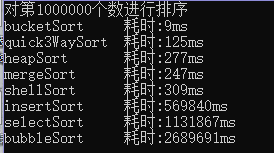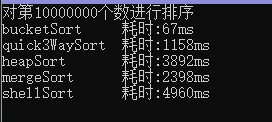总结：
1.在这8中排序算法当中，桶排序是最快的，冒泡排序最慢。
但是，桶排序要求构建一个待排序最大元素的数组，往往排序的时候我们并不能确定最大元素的值，另一方面和并归排序一样，当数组很大的时候，也要考虑额外的内存分配问题。
2.在数组非常长的排序，各种排序算法中，三向切分快速排序是最快的（较快速排序提升了20%到30%）。但是，需要考虑递归产生临时是否会导致栈溢出的问题。堆排序时间复杂度ONlogN,空间复杂度1，并且对已经排序好的数组插入一个元素的时候，堆是一种优先队列的时间，查找和插入的时间复杂度较优。
3.对于小数组，快速排序比插入排序慢。因此在设计排序的时候可以考虑通过判断长度来切换排序算法。

展开全文排序算法
• 输入: 一最多包含n不重复的正整数的文件，其中每个数都小于n，每个数是一7位的整数, n=10^7。 条件: 最多有1MB的内存可用, 排序最多只允许执行几分钟,10s是比较理想的运行时间.有充足的磁盘存储空间可用. ...磁盘排序
• # __author__ ='wuwa'# -*- coding: utf-8 -*-import random'''随机生成10010至1000之间的生成的100个数进行排序，禁止使用Python自带的排序函数，要自己实现排序函数'''class MySort:# 生成随机数,返回排序...
• 排序，顾名思义，会用到“桶”，核心思想是将要排序的数据分到几有序的桶里，每桶里的数据再单独进行排序。桶内排完序之后，再把每桶里的数据按照顺序依次取出，组成的序列就是有序的了。比如说我们有10GB的...数据结构 排序算法 快速排序
• 前言：干货干货，作者偶然在工作中悟出来了一种排序算法，听标题就很牛逼，下面开始一一...2、用1000个升序数据进行排序，小于几毫秒我就不写了，直接写毫秒 C++Sort时间为：2ms，快排：2ms，波浪：1ms，桶排1ms,桶...排序 快速排序
• 然而，有一种任务，例如，超过1000万个元素的数组进行排序，这种任务本身可以并发执行，但如何拆解成小任务需要在任务执行的过程中动态拆分。这样，大任务可以拆成小任务，小任务还可以继续拆成更小的任务，最后把...多线程求和
• 在100万级大数据量插入，MongoDB中速度还相当快的，下面分享插入1000万条数据测试结果。 下面分享Java操作代码 @Test void saveBatch() { long start=System.currentTimeMillis(); int oneNum=5000; List<...
• 最多1000万个正整数的文件，每个数7位正整数，没有任何重复，不与其他关联  输出： 升序排列的输出文件 约束：1MB的内存空间，最短的运行时间 1000万数据需要使用800万位（1MB）来表示，只使用一次读入读出文件。...
• #这程序是把以下几个排序全部执行并输出各自的排序时间 import time import random class Sort: # 快速排序 def parttion(a, low, high): pivotkey = a[low] while (low < high): # 因为得把所有元素都按...python 排序算法
• 首先想到的是使用快速排序先将元素排好序，再取出最大的那一部分1000个数，由于快速排序的时间复杂度为o(nlog2n)，也就是(10^ 8)× log2(10^ 8)，约等于(10^ 8)× 27，这也是非常大的操作量了。由于快速排序是原地...数据结构 快速排序
• 1000万数据怎么同时需要排序和持续记录，用什么语句或者思路实现？既要实现实时的数据记录，也要能保持数据的排序顺序？
• 题目：随机生成100小于1000，从大到小排序，并输出次最大值。 拿到题目的时候，想的好简单，冒泡排序，从大到小，取数组中第二个数，就是次最大值。回来又细想了下，如果随机生成的中有重复值，这么做就...java 冒泡排序
• ## C++：六种排序算法的时间比较

千次阅读 多人点赞 2020-08-08 12:27:44
/* 说明：以下排序算法将分别对1000、1、10、100万个随机数进行排序，记录排序所需时间，并进行比较。VS2017 */ 1.冒泡排序法：平均时间复杂度：O（n^2） #include<iostream> #include<time.h> #...排序算法 快速排序
• 星期天（2012.5.6）中午去华科参加了百度的笔试，试卷的最后一题是问百度搜索框的suggestion提示功能如何实现，用什么数据结构和算法。 我简单地提及了一下Top K。 前段时间看过算法大牛JULY博客中的一些面试...
• 测试数据为随机生成，可设置为10万、100万、1000万大小的数组。在代码中提供了详细的注释，在容易出错的地方进行了解释。下面是得到的输出结果。 the array num is :1000000 The mergesort run time is:15931ms! The...
• 归并排序充分利用递归...//归并排序，两两递归的试一半一的分隔直接到只有一元素时返回，然后两两的有序数组进行归并排序，效率相当高 public class MergeSort { /** * @param sourceArray 要排序的源数据 ...java 归并排序
• 本书第一章提出了一看似简单的问题，有最多1000万条不同的整型数据存在于硬盘的文件中，如何在1M内存的情况下其进行尽可能快的排序。 每数字用4byte，1M即可存储250 000数据，显然，只要每次250 000...
• 在工作中我们常遇到此类问题，从一大量甚至海量的数据中取出前几大的。必须在海量的文章中取出点击量最大的10篇文章。此类问题其实就是Top K问题。给定一数据(数据量海量 N)，想找到前 K 最大的或最小的...
• 1. 100数据的产生,随机数方式 #include #include #include #include #include using namespace std; const int size = 10000000; int num[size]; int main() { int n; FILE *fp = fopen("data.txt", "w");
• 10亿个数中找出1000个最大的的算法思路： 1，先拿出前1000个数字...3, 如果tempMaxValue 比 minValue 大， 则将 theMaxValue 放入前1000个数中,再排序并找出minValue . 4 … 依此类推。 即可得到1000个最大的。...
• (2) (b,d]重复(1)操作，直到最右边的区间个数小于100。注意[a,b)区间不用划分  (3) 返回上一区间，并返回此区间的数字数目。接着方法仍然是上一区间的左边进行划分，分为[a2,b2）b2（b2,d2]两区间，取
• 在大规模数据处理中，经常会遇到的一类问题：在海量数据中找出出现频率最好的前k个数，...该方法并不高效，因为题目的目的是寻找出最大的10000个数即可，而排序却是将所有的元素都排序了，做了很多的无用功。 2、...
• 10种常见排序算法的原理，包括冒泡排序、选择排序、插入排序、希尔排序、堆排序、归并排序、快速排序、计数排序、桶排序、基数排序。并且每种排序都提供了Java代码的实现案例。java 排序算法 堆排序 快速排序 冒泡排序
• 随机生成10w随机数，排序，测时间------双向冒泡排序+'哨兵'边界排序算法
• 如何在1亿个数中找出最大的100个数（top K问题） ​ 最容易想到的方法是将...其实即使内存能够满足要求（我机器内存都是8GB），该方法也并不高效，因为题目的目的是寻找出最大的10000个数即可，而排序却是将所有的元素数据结构...